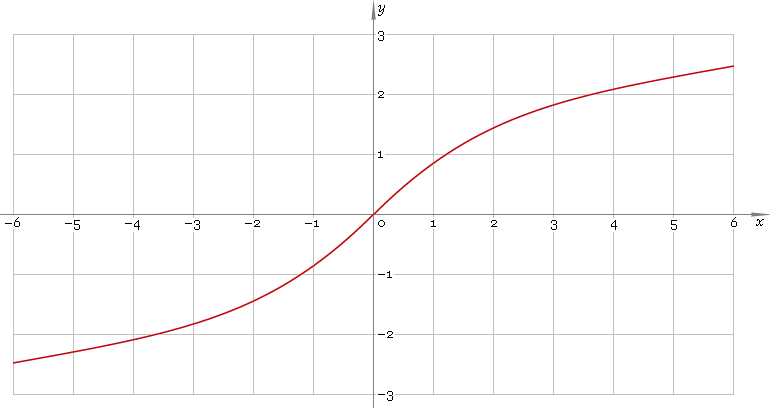The Art of Mathematics

# arsinh or arsh — arc-hyperbolic sine function

## 1. Definition

Arc-hyperbolic sine is inverse of hyperbolic sine function.

arsinhxsinhinvx

With the help of natural logarithm it can be represented as:

arsinhx ≡ ln[x + √(x2 + 1)]

## 2. Graph

Arc-hyperbolic sine is antisymmetric function defined everywhere on real axis. Its graph is depicted below — fig. 1.Fig. 1. Graph of the arc-hyperbolic sine function y = arsinhx.

Function codomain is entire real axis.

## 3. Identities

Property of antisymmetry:

arsinh−x = −arsinhx

Reciprocal argument:

arsinh(1/x) = arcschx

Sum and difference:

arsinhx + arsinhy = arsinh[x√(y2 + 1) + y√(x2 + 1)]
arsinhx − arsinhy = arsinh[x√(y2 + 1) − y√(x2 + 1)]
arsinhx + arcoshy = arsinh{xy + √[(x2 + 1)(y2 − 1)]} = arcosh[y√(x2 + 1) + x√(y2 − 1)]

## 4. Derivative and indefinite integral

Arc-hyperbolic sine derivative:

arsinh′x = 1 /√(1 + x2)

Indefinite integral of the arc-hyperbolic sine:

∫ arsinhx dx = x arsinhx − √(1 + x2) + C

where C is an arbitrary constant.

## 5. How to use

To calculate arc-hyperbolic sine of the number:

``arsinh(−1);``

To get arc-hyperbolic sine of the complex number:

``arsinh(−1+i);``

To get arc-hyperbolic sine of the current result:

``arsinh(rslt);``

To get arc-hyperbolic sine of the number z in calculator memory:

``arsinh(mem[z]);``

## 6. Support

Arc-hyperbolic sine of the real argument is supported in free version of the Librow calculator.

Arc-hyperbolic sine of the complex argument is supported in professional version of the Librow calculator.